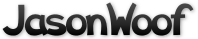Got questions, comments, patches, etc.? Contact Jason Woofenden
7b6286732228e755ee2fae7880cb33def25ec3c8
1 #include <SDL.h>
2 #include <math.h>
4 #include "config.h"
5 #include "globals.h"
6 #include "dust.h"
7 #include "mt.h"
9 struct dust_mote {
10         float x,y,z;
11         Uint16 color;
12 };
14 struct dust_mote motes[N_DUST_MOTES];
16 void
17 init_dust(void)
18 {
19         int i, b;
20         for(i=0; i<N_DUST_MOTES; i++) {
21                 motes[i].x = frnd()*(XSIZE-5);
22                 motes[i].y = frnd()*(YSIZE-5);
23                 motes[i].z = MAX_DUST_DEPTH*sqrt(frnd());
24                 b = (MAX_DUST_DEPTH - motes[i].z) * 255.0 / MAX_DUST_DEPTH;
25                 motes[i].color = SDL_MapRGB(surf_screen->format, b, b, b);
26         }
27 }
29 void
30 move_dust(float ticks)
31 {
32         int i;
33         float xscroll = screendx * ticks;
34         float yscroll = screendy * ticks;
36         // Originally this code was much simpler, but it would crash sometimes
37         // because the floating point numbers wouldn't always round the same
38         // direction, and we'd ocanially try to draw off the screen.
40         for(i=0; i<N_DUST_MOTES; i++) {
41                 motes[i].x -= xscroll / (1.3 + motes[i].z);
42                 motes[i].y -= yscroll / (1.3 + motes[i].z);
44                 if(motes[i].x < 0) {
45                         motes[i].x += XSIZE;
46                 }
47                 if(motes[i].x > (XSIZE - 0.000001)) {
48                         motes[i].x -= XSIZE;
49                         if(motes[i].x < 0) {
50                                 motes[i].x = 0;
51                         }
52                 }
54                 if(motes[i].y < 0) {
55                         motes[i].y += YSIZE;
56                 }
57                 if(motes[i].y > (YSIZE - 0.000001)) {
58                         motes[i].y -= YSIZE;
59                         if(motes[i].y < 0) {
60                                 motes[i].y = 0;
61                         }
62                 }
63         }
64 }
66 void
67 draw_dust(SDL_Surface *s)
68 {
69         int i;
70         uint16_t *pixels = s->pixels;
71         for(i=0; i<N_DUST_MOTES; i++) {
72                 pixels[s->pitch/2*(int)motes[i].y + (int)motes[i].x] = motes[i].color;
73         }
74 }